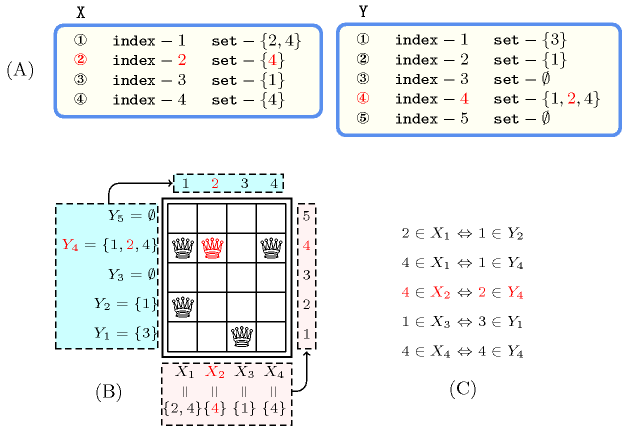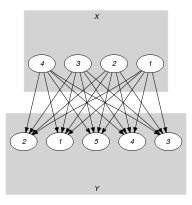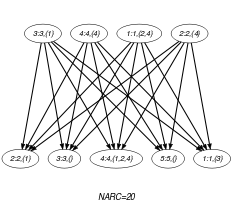## 5.201. inverse_set

Origin
Constraint

$\mathrm{𝚒𝚗𝚟𝚎𝚛𝚜𝚎}_\mathrm{𝚜𝚎𝚝}\left(𝚇,𝚈\right)$

Arguments
 $𝚇$ $\mathrm{𝚌𝚘𝚕𝚕𝚎𝚌𝚝𝚒𝚘𝚗}\left(\mathrm{𝚒𝚗𝚍𝚎𝚡}-\mathrm{𝚒𝚗𝚝},\mathrm{𝚜𝚎𝚝}-\mathrm{𝚜𝚟𝚊𝚛}\right)$ $𝚈$ $\mathrm{𝚌𝚘𝚕𝚕𝚎𝚌𝚝𝚒𝚘𝚗}\left(\mathrm{𝚒𝚗𝚍𝚎𝚡}-\mathrm{𝚒𝚗𝚝},\mathrm{𝚜𝚎𝚝}-\mathrm{𝚜𝚟𝚊𝚛}\right)$
Restrictions
 $\mathrm{𝚛𝚎𝚚𝚞𝚒𝚛𝚎𝚍}$$\left(𝚇,\left[\mathrm{𝚒𝚗𝚍𝚎𝚡},\mathrm{𝚜𝚎𝚝}\right]\right)$ $\mathrm{𝚛𝚎𝚚𝚞𝚒𝚛𝚎𝚍}$$\left(𝚈,\left[\mathrm{𝚒𝚗𝚍𝚎𝚡},\mathrm{𝚜𝚎𝚝}\right]\right)$ $\mathrm{𝚒𝚗𝚌𝚛𝚎𝚊𝚜𝚒𝚗𝚐}_\mathrm{𝚜𝚎𝚚}$$\left(𝚇,\mathrm{𝚒𝚗𝚍𝚎𝚡}\right)$ $\mathrm{𝚒𝚗𝚌𝚛𝚎𝚊𝚜𝚒𝚗𝚐}_\mathrm{𝚜𝚎𝚚}$$\left(𝚈,\mathrm{𝚒𝚗𝚍𝚎𝚡}\right)$ $𝚇.\mathrm{𝚒𝚗𝚍𝚎𝚡}\ge 1$ $𝚇.\mathrm{𝚒𝚗𝚍𝚎𝚡}\le |𝚇|$ $𝚈.\mathrm{𝚒𝚗𝚍𝚎𝚡}\ge 1$ $𝚈.\mathrm{𝚒𝚗𝚍𝚎𝚡}\le |𝚈|$ $𝚇.\mathrm{𝚜𝚎𝚝}\ge 1$ $𝚇.\mathrm{𝚜𝚎𝚝}\le |𝚈|$ $𝚈.\mathrm{𝚜𝚎𝚝}\ge 1$ $𝚈.\mathrm{𝚜𝚎𝚝}\le |𝚇|$
Purpose

The following two statements are equivalent:

1. Value $j$ belongs to the set variable of the ${i}^{th}$ item of the $𝚇$ collection.

2. Value $i$ belongs to the set variable of the ${j}^{th}$ item of the $𝚈$ collection.

I.e., $j\in 𝚇\left[i\right]⇔i\in 𝚈\left[j\right]$.

Example
$\left(\begin{array}{c}〈\begin{array}{cc}\mathrm{𝚒𝚗𝚍𝚎𝚡}-1\hfill & \mathrm{𝚜𝚎𝚝}-\left\{2,4\right\},\hfill \\ \mathrm{𝚒𝚗𝚍𝚎𝚡}-2\hfill & \mathrm{𝚜𝚎𝚝}-\left\{4\right\},\hfill \\ \mathrm{𝚒𝚗𝚍𝚎𝚡}-3\hfill & \mathrm{𝚜𝚎𝚝}-\left\{1\right\},\hfill \\ \mathrm{𝚒𝚗𝚍𝚎𝚡}-4\hfill & \mathrm{𝚜𝚎𝚝}-\left\{4\right\}\hfill \end{array}〉,\hfill \\ 〈\begin{array}{cc}\mathrm{𝚒𝚗𝚍𝚎𝚡}-1\hfill & \mathrm{𝚜𝚎𝚝}-\left\{3\right\},\hfill \\ \mathrm{𝚒𝚗𝚍𝚎𝚡}-2\hfill & \mathrm{𝚜𝚎𝚝}-\left\{1\right\},\hfill \\ \mathrm{𝚒𝚗𝚍𝚎𝚡}-3\hfill & \mathrm{𝚜𝚎𝚝}-\varnothing ,\hfill \\ \mathrm{𝚒𝚗𝚍𝚎𝚡}-4\hfill & \mathrm{𝚜𝚎𝚝}-\left\{1,2,4\right\},\hfill \\ \mathrm{𝚒𝚗𝚍𝚎𝚡}-5\hfill & \mathrm{𝚜𝚎𝚝}-\varnothing \hfill \end{array}〉\hfill \end{array}\right)$

The $\mathrm{𝚒𝚗𝚟𝚎𝚛𝚜𝚎}_\mathrm{𝚜𝚎𝚝}$ constraint holds since:

$\left\{\begin{array}{cc}2\in 𝚇\left[1\right].\mathrm{𝚜𝚎𝚝}⇔1\in 𝚈\left[2\right].\mathrm{𝚜𝚎𝚝},\hfill & 4\in 𝚇\left[1\right].\mathrm{𝚜𝚎𝚝}⇔1\in 𝚈\left[4\right].\mathrm{𝚜𝚎𝚝},\hfill \\ 4\in 𝚇\left[2\right].\mathrm{𝚜𝚎𝚝}⇔2\in 𝚈\left[4\right].\mathrm{𝚜𝚎𝚝},\hfill \\ 1\in 𝚇\left[3\right].\mathrm{𝚜𝚎𝚝}⇔3\in 𝚈\left[1\right].\mathrm{𝚜𝚎𝚝},\hfill \\ 4\in 𝚇\left[4\right].\mathrm{𝚜𝚎𝚝}⇔4\in 𝚈\left[4\right].\mathrm{𝚜𝚎𝚝}.\hfill \end{array}\right\$

Typical
 $|𝚇|>1$ $|𝚈|>1$
Symmetries
• Arguments are permutable w.r.t. permutation $\left(𝚇,𝚈\right)$.

• Items of $𝚇$ are permutable.

• Items of $𝚈$ are permutable.

Usage

The $\mathrm{𝚒𝚗𝚟𝚎𝚛𝚜𝚎}_\mathrm{𝚜𝚎𝚝}$ constraint can for instance be used in order to model problems where one has to place items on a rectangular board in such a way that a column or a row can have more than one item. We have one set variable for each row of the board; Its values are the column indexes corresponding to the positions where an item is placed. Similarly we have also one set variable for each column of the board; Its values are the row indexes corresponding to the positions where an item is placed. The $\mathrm{𝚒𝚗𝚟𝚎𝚛𝚜𝚎}_\mathrm{𝚜𝚎𝚝}$ constraint maintains the link between the rows and the columns variables. Figure 5.201.1 shows the board that can be associated with the example of the Example slot.

##### Figure 5.201.1. Illustration of the Example slot where we highlight in red the second item of the $𝚇$ collection and the fourth item of the $𝚈$ collection showing the relation between ${X}_{2}$ and ${Y}_{4}$, where ${X}_{i}$ (with $1\le i\le 4$) and ${Y}_{j}$ (with $1\le j\le 5$) respectively stands for the set attribute of the ${i}^{\mathrm{𝚝𝚑}}$ item of the $𝚇$ collection and of the ${j}^{\mathrm{𝚝𝚑}}$ item of the $𝚈$ collection (A) Collections $𝚇$ and $𝚈$ passed to the $\mathrm{𝚒𝚗𝚟𝚎𝚛𝚜𝚎}_\mathrm{𝚜𝚎𝚝}$ constraint, (B) Corresponding board, (C) Conditions linking the items of $𝚇$ and the items of $𝚈$.Systems

specialisation: $\mathrm{𝚒𝚗𝚟𝚎𝚛𝚜𝚎}$ ($\mathrm{𝚜𝚎𝚝}$ $\mathrm{𝚟𝚊𝚛𝚒𝚊𝚋𝚕𝚎}$ replaced by $\mathrm{𝚍𝚘𝚖𝚊𝚒𝚗}\mathrm{𝚟𝚊𝚛𝚒𝚊𝚋𝚕𝚎}$).

Keywords
Arc input(s)

$𝚇$ $𝚈$

Arc generator
$\mathrm{𝑃𝑅𝑂𝐷𝑈𝐶𝑇}$$↦\mathrm{𝚌𝚘𝚕𝚕𝚎𝚌𝚝𝚒𝚘𝚗}\left(𝚡,𝚢\right)$

Arc arity
Arc constraint(s)
$\mathrm{𝚒𝚗}_\mathrm{𝚜𝚎𝚝}$$\left(𝚢.\mathrm{𝚒𝚗𝚍𝚎𝚡},𝚡.\mathrm{𝚜𝚎𝚝}\right)⇔$$\mathrm{𝚒𝚗}_\mathrm{𝚜𝚎𝚝}$$\left(𝚡.\mathrm{𝚒𝚗𝚍𝚎𝚡},𝚢.\mathrm{𝚜𝚎𝚝}\right)$
Graph property(ies)
$\mathrm{𝐍𝐀𝐑𝐂}$$=|𝚇|*|𝚈|$

Graph model

Parts (A) and (B) of Figure 5.201.2 respectively show the initial and final graph associated with the Example slot. Since we use the $\mathrm{𝐍𝐀𝐑𝐂}$ graph property, the arcs of the final graph are stressed in bold.

##### Figure 5.201.2. Initial and final graph of the $\mathrm{𝚒𝚗𝚟𝚎𝚛𝚜𝚎}_\mathrm{𝚜𝚎𝚝}$ constraint(a) (b)